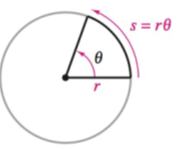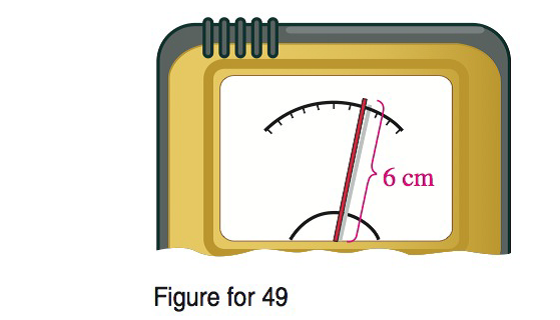Chapter 8.1, Problem 49E### Calculus: An Applied Approach (Min...

10th Edition
Ron Larson
ISBN: 9781305860919

#### Solutions

Chapter
Section### Calculus: An Applied Approach (Min...

10th Edition
Ron Larson
ISBN: 9781305860919
Textbook Problem
1 views

# Arc Length In Exercises 47-50, use the following information, as shown in the figure. For a circle of radius r, a central angle θ (in radians) intercepts an arc of length s given by s = r θ .Instrumentation The pointer of a voltmeter is 6 centimeters in length (see figure). Find the angle (in radians and degrees) through which the pointer rotates when it moves 2.5 centimeters on the scale.To determine

To calculate: The angle moved by pointer of the voltmeter in both radians and in degrees if rotation of pointer on the scale is 2.5 cm, and its length is 6 cm.

Explanation

Given Information:

The rotation of pointer on the scale is 2.5 cm and its length is 6 cm. The figure provided below shows the pointer of a voltmeter.

Formula used:

The length of an arc s of a circle of radius r is s=rθ, where θ is the central angle in radians.

In order to convert the angle from radians to degrees, multiply the angle by 180°π radians .

Calculation:

Consider the length of arc, s=rθ

The angle θ is in radians.

The rotation of pointer s on the scale is 2.5 cm and its length r is 6 cm.

Substitute s=2.5 cm and r=6 cm in the equation s=rθ.

2.5 cm=(6 cm)θθ=2

### Still sussing out bartleby?

Check out a sample textbook solution.

See a sample solution

#### The Solution to Your Study Problems

Bartleby provides explanations to thousands of textbook problems written by our experts, many with advanced degrees!

Get Started

#### Find more solutions based on key concepts